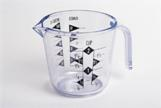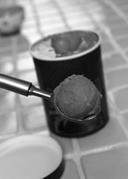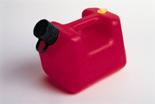## Units of Capacity

### LEARNING outcomes

• Define units of length and convert from one to another.
• Perform arithmetic calculations on units of length.
• Solve application problems involving units of length.
• Define units of weight and convert from one to another.
• Perform arithmetic calculations on units of weight.
• Solve application problems involving units of weight.
• Describe the general relationship between the U.S. customary units and metric units of length, weight/mass, and volume.
• Define the metric prefixes and use them to perform basic conversions among metric units.
• Solve application problems involving metric units of length, mass, and volume.
• State the freezing and boiling points of water on the Celsius and Fahrenheit temperature scales.
• Convert from one temperature scale to the other, using conversion formulas.

Capacity is the amount of liquid (or other pourable substance) that an object can hold when its full. When a liquid, such as milk, is being described in gallons or quarts, this is a measure of capacity.

Understanding units of capacity can help you solve problems like this: Sven and Johanna were hosting a potluck dinner. They did not ask their guests to tell them what they would be bringing, and three people ended up bringing soup. Erin brought 1 quart, Richard brought 3 pints, and LeVar brought 9 cups. How many cups of soup did they have all together?

There are five main units for measuring capacity in the U.S. customary measurement system. The smallest unit of measurement is a fluid ounce. Ounce is also used as a measure of weight, so it is important to use the word fluid with ounce when you are talking about capacity. Sometimes the prefix fluid is not used when it is clear from the context that the measurement is capacity, not weight.

The other units of capacity in the customary system are the cup, pint, quart, and gallon. The table below describes each unit of capacity and provides an example to illustrate the size of the unit of measurement.

 Fluid Ounce A unit of capacity equal to $\displaystyle \frac{1}{8}$ of a cup. One fluid ounce of water at 62°F weighs about one ounce. The amount of liquid medicine is often measured in fluid ounces.Cup A unit equal to 8 fluid ounces. The capacity of a standard measuring cup is one cup.Pint A unit equal to 16 fluid ounces, or 2 cups. The capacity of a carton of ice cream is often measured in pints.Quart A unit equal to 32 fluid ounces, or 4 cups. You often see quarts of milk being sold in the supermarket.Gallon A unit equal to 4 quarts, or 128 fluid ounces. When you fill up your car with gasoline, the price of gas is often listed in dollars per gallon.You can use any of these five measurement units to describe the capacity of an object, but it makes more sense to use certain units for certain purposes. For example, it makes more sense to describe the capacity of a swimming pool in gallons and the capacity of an expensive perfume in fluid ounces.

### Try It

Sometimes you will need to convert between units of measurement. For example, you might want to express 5 gallons of lemonade in cups if you are trying to determine how many 8-fluid ounce servings the amount of lemonade would yield.

The table below shows some of the most common equivalents and conversion factors for the five customary units of measurement of capacity.

 Unit Equivalents Conversion Factors (heavier to lighter units of measurement) Conversion Factors (lighter to heavier units of measurement) 1 cup = 8 fluid ounces $\displaystyle \frac{\text{1 cup}}{\text{8 fluid ounces}}$ $\displaystyle \frac{\text{8 fluid ounces}}{\text{1 cup}}$ 1 pint = 2 cups $\displaystyle \frac{\text{1 pint}}{2\text{ cups}}$ $\displaystyle \frac{2\text{ cups}}{1\text{ pint}}$ 1 quart = 2 pints $\displaystyle \frac{\text{1 quart}}{2\text{ pints}}$ $\displaystyle \frac{2\text{ pints}}{\text{1 quart}}$ 1 quart = 4 cups $\displaystyle \frac{\text{1 quart}}{4\text{ cups}}$ $\displaystyle \frac{4\text{ cups}}{\text{1 quart}}$ 1 gallon = 4 quarts $\displaystyle \frac{\text{1 gallon}}{4\text{ quarts}}$ $\displaystyle \frac{4\text{ quarts}}{\text{1 gallon}}$ 1 gallon = 16 cups $\displaystyle \frac{1\text{ gallon}}{\text{16 cups}}$ $\displaystyle \frac{\text{16 cups}}{1\text{ gallon}}$

## Converting Between Units of Capacity

As with converting units of length and weight, you can use the factor label method to convert from one unit of capacity to another. An example of this method is shown below.

### Example

How many pints is $\displaystyle 2\frac{3}{4}$ gallons?

### Example

How many gallons is 32 fluid ounces?

### Try It

Find the sum of 4 gallons and 2 pints. Express your answer in cups.

## Applying Unit Conversions

There are times when you will need to combine measurements that are given in different units. In order to do this, you need to convert first so that the units are the same.

Consider the situation posed earlier in this topic.

### Exercises

Sven and Johanna were hosting a potluck dinner. They did not ask their guests to tell them what they would be bringing, and three people ended up bringing soup. Erin brought 1 quart, Richard brought 3 pints, and LeVar brought 9 cups. How much soup did they have total?

### Exercises

Natasha is making lemonade to bring to the beach. She has two containers. One holds one gallon and the other holds 2 quarts. If she fills both containers, how many cups of lemonade will she have?

Another way to work the problem above would be to first change 1 gallon to 16 cups and change 2 quarts to 8 cups. Then add: $16+8=24$ cups.

In the following video we provide another example of using unit conversions to solve a problem.  We show how to find the number of lemons needed to make a pie, given that each lemon yields about 4 tablespoons of juice.

### Try It

Alan is making chili. He is using a recipe that makes 24 cups of chili. He has a 5-quart pot and a 2-gallon pot and is trying to determine whether the chili will all fit in one of these pots. Which of the pots will fit the chili?

In the following ~10 minute video, we provide a mini-lesson that covers US measurements for length, weight, and capacity, and how to convert between larger and smaller units for each type. This is a good summary of the concepts covered in the US Units of Measurement section of this module.

## Summary

There are five basic units for measuring capacity in the U.S. customary measurement system. These are the fluid ounce, cup, pint, quart, and gallon. These measurement units are related to one another, and capacity can be described using any of the units. Typically, people use gallons to describe larger quantities and fluid ounces, cups, pints, or quarts to describe smaller quantities. Often, in order to compare or to solve problems involving the amount of liquid in a container, you need to convert from one unit of measurement to another.